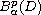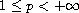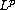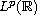Electron. J. Differential Equations, Vol. 2017 (2017), No. 236, pp. 1-8.

### Heat and Laplace type equations with complex spatial variables in weighted Bergman spaces Ciprian G. Gal, Sorin G. Gal

Abstract:
In a recent book, the authors of this paper have studied the classical heat and Laplace equations with real time variable and complex spatial variable by the semigroup theory methods, under the hypothesis that the boundary function belongs to the space of analytic functions in the open unit disk and continuous in the closed unit disk, endowed with the uniform norm. The purpose of the present note is to show that the semigroup theory methods works for these evolution equations of complex spatial variables, under the hypothesis that the boundary function belongs to the much larger weighted Bergman spacewith, endowed with a-norm. Also, the case of several complex variables is considered. The proofs require some new changes appealing to Jensen's inequality, Fubini's theorem for integrals and the-integral modulus of continuity. The results obtained can be considered as complex analogues of those for the classical heat and Laplace equations inspaces.

Submitted August 25, 2017. Published September 29, 2017.
Math Subject Classifications: 47D03, 47D06, 47D60.
Key Words: Complex spatial variable; semigroups of linear operators; heat equation; Laplace equation; weighted Bergman space.

Show me the PDF file (202 KB), TEX file for this article.Ciprian G. Gal Department of Mathematics Florida International University Miami, FL 33199, USA email: cgal@fiu.edu Sorin G. Gal University of Oradea Department of Mathematics and Computer Science Str. Universitatii Nr. 1 410087 Oradea, Romania email: galso@uoradea.ro